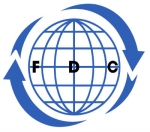Search all upcoming seminars, conferences, short management courses and training in Nigeria and around the WorldData Analysis, Modeling and Simulation using Excel Course

By: Foscore Development Center

Kenya

14 - 18 Mar, 2022  5 days

USD 1,000

Venue: Foscore Development Training Center, Nairobi

Other Dates

Venue Date Fee
Foscore Development Training Center, Nairobi, Kenya 06 - 10 Jun, 2022 USD1000
Foscore Development Training Center, Nairobi, Kenya 29 Aug - 02 Sep, 2022 USD1000
Foscore Development Training Center, Nairobi, Kenya 21 - 25 Nov, 2022 USD1000

This course is about Statistics and Data Analysis. The course will teach you the advanced concepts related to Statistics and Data Analysis and help you in applying these concepts. Various examples and datasets will be used and then we will show you how to use Microsoft Excel to perform these calculations.

Course Objective:

• Descriptive and Inferential Statistics.
• Statistical calculations.
• Understand statistics from basic to advance using excel

Who should attend?

Statistician, analyst, or a budding data scientist and beginners who want to learn how to analyze data with excel.

Course content

• Statistics Introduction and Definitions
• Types of Data in Excel - Text, Numbers, Date/Time, Logical
• Qualitative vs Quantitative Data / Continuous vs Discrete
• Nominal, Ordinal, Interval and Ratio
• Population and Sample (Theory)
• Understanding Data Types
• Understanding Formulas and Functions
• Relative vs Absolute Reference
• Formulas, Functions and Named Ranges
• Descriptive Statistics
• Section Introduction - Descriptive Statistics
• Descriptive Statistics - Central Tendency
• Descriptive Statistics - Variation
• Installing Data Analysis Pack and Calculating Descriptive Statistics
• Descriptive Statistics - Central Tendency
• Descriptive Statistics - Variation (Excel)
• Descriptive Statistics - Shapes
• Descriptive Statistics
• Data Visualization
• Three commonly used Charts
• Create Histogram
• Histogram Shapes
• Create Box and Whisker Plot
• Scatter Diagram
• Create Scatter Plot
• Data Visualization
• Introduction to Basic Probability Concepts
• Basic Probability Concepts
• Calculate Factorial, Permutations and Combinations
• Basic Probability Concepts
• Probability Distributions
• Central Limit Theorem Demonstration
• Normal Probability Distribution
• Binomial Probability Distribution
• Other Distributions Related to Binomial Distribution
• Poisson Distribution
• Probability Distributions
• Introduction Hypothesis Testing
• Hypothesis Testing - Types of Errors
• Hypothesis Testing Explained
• Tests for Mean, Variance and Proportions
• One Sample z Test
• Two Tail Example
• Understanding the p Value
• Introduction to T Functions
• One Sample t Test - Example
• One Sample p Test
• Two Sample z Test
• Two Sample t Test - Equal Variance
• Two Sample t Test - Unequal Variance
• Two Sample t Test - Paired t Test
• Two Sample p Test
• Introduction Tests for Variance (F and Chi Square)
• F Test to Compare Variances
• Plotting F Distribution
• F Distribution Related Functions
• F.TEST Function
• F Test Using Data Analysis Pack
• Chi Square Test for Variance
• Plotting Chi Square Distribution
• Chi Square Distribution Related Functions
• Chi Square Test
• Introduction to ANOVA
• Understanding the concept of ANOVA
• Formulas and calculations in ANOVA
• Performing ANOVA
• Two Factor ANOVA without Replication
• Two Factor ANOVA with Replication
• Goodness of Fit and Contingency Table
• Introduction Goodness of Fit and Contingency Tables
• Goodness of Fit Test
• Contingency Table
• Correlation and Linear Regression
• Introduction to Correlation
• Plotting Scatter Plot and Calculating Correlation Coefficient
• Correlation Coefficient for Multiple Variables
• Correlation Related Functions
• Correlation vs Causation
• Coefficient of Determination
• Manual Calculation
• Slope, Intercept
• Forecast, Linear, Trend and Lines
• Regression using Data Analysis Pack

Note

• All our courses can be Tailor-made to participants needs
• Course duration is flexible and the contents can be modified to fit any number of days.
• Presentations are well guided, practical exercise, web based tutorials and group work. Our facilitators are expert with more than 10years of experience.
• One year free Consultation and Coaching provided after the course.
 Foscore Development Training Center, Nairobi Mar 14 - 18 Mar, 2022 Foscore Development Training Center, Nairobi, Kenya 06 - 10 Jun, 2022 Foscore Development Training Center, Nairobi, Kenya 29 Aug - 02 Sep, 2022 Foscore Development Training Center, Nairobi, Kenya 21 - 25 Nov, 2022
 USD 1,000.00
(Convert Currency)

Jackson Munene +254 712260031

A discount of 20% for 5 or more participants
Foscore Development Center

Related CoursesQuantitative Data Management and Analysis with R Course

5 days, 28 Mar - 01 Apr, 2022

Foscore Development Center

This course is designed for participants who plan to use R for the management, coding, analysis and visualization of qualitative data. The course’s content is spread over seven modules and ...Advanced Project Management Course

26 days, 21 Feb - 18 Mar, 2022

Foscore Development Center

Successfully managing a project requires effective planning and adherence to the laid down practices in every step of the process. By understanding project management, you will be better prepared to ...Rural Development Course

5 days, 21 - 25 Feb, 2022

Foscore Development Center

This training guide has been designed for use in introductory rural and microfinance training courses. While the fundamentals of development finance and approaches in microfinance apply to rural ...Research Design,ODK Mobile Data Collection,GIS Mapping,Data Analysis using NVIVO and PYTHON Course

12 days, 31 Jan - 11 Feb, 2022

Foscore Development Center

New developments in data science offer a tremendous opportunity to improve decision-making. In the development world, there has been an increase in the number of data gathering initiative such as ...